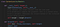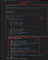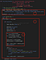# ATD Logo, Picasso Style: Use Convolutional Neural Network to Transfer Painting Styles (PyTorch)

At ATD, we deploy machine learning solutions to drive impacts to the business. Even cutting-edge technologies such as deep learning, which is extremely popular in the fields of robotics, autonomous driving and natural language processing, can be utilized to help traditional industry problems in logistics, supply chain management, and inventory control.

For instance, we utilize recurrent neural networks (RNN) to solve problems with our staffing activity forecasting models and optimizing our inventory efficiencies across our supply chain. We are also developing Convolutional Neural Networks (CNN) to facilitate automatic tire counting and labeling as well. These Neural Networks are incredibly useful to ATD, but how do they actually work?

A CNN is a class of deep, feed-forward artificial neural networks, most commonly applied to analyzing visual imagery, which has become a key driver of Deep Learning’s rise to fame. From autopilot in the new Tesla cars, to photos taken with the Pixel 3 smartphone, CNN is running behind the scenes, making magic happen every day.

The actual CNN algorithm is surprisingly intuitive. In fact, the human brain follows a similar process when identifying dots, edges and patterns to identify images from human sight. CNNs have layers of computational “neurons” that find dots, edges, and patterns: those are essentially the “style” of an image.

But CNNs can go beyond the capabilities of the human brain. CNNs are so accurate, that they can find cancerous cells from medical imaging better than humans. If we can take a trained CNN’s first few convolutional layers, and project the patterns to another image, couldn’t we fuse the style of one painting with the content of another painting?

To put it simply, we want to use a pre-trained CNN and extract the content information from an image p and the style information from another image q and somehow combine p’s content and q’s style together.

More specifically, to get an accurate representation of the image p’s content, we formulate a loss of content, L_content(p,x), where p is the original input content image, and x is some white noise input image. By minimizing the L_content(p,x), we are essentially reforming x, so that it goes from white noise to something that looks like p, in terms of content.

To get an accurate representation of the image q style, we formulate a loss of style, L_style(q,x), where q is the original input style image, and x again is some white noise input image. By minimizing the L_style(q,x), we are reforming x to have similar “styles” of image q.

So, how exactly do we compute content loss L_content(p,x)? If you really think about it, as the content image p passes through convolutional layers and pooling layers, its content or pixels are being filtered through filters and max poolers, and eventually end up in some feature spaces. If we can morph the white noise image x to a similar position close to p in the feature space, then the white noise image x must have similar content or pixels.

So, let’s say for a given convolutional layer N_l, it has m filters, then we can flatten each filter’s 2D features as 1D vectors of length n (filter height * filter width); so, for convolutional layer N_l, the content of image p can be represented as its feature space values F(l,p). Similarly, when we pass white noise image x through N_l, its content has feature values of F(l,x). Note, F(l, . ) is a matrix of dimension m * n.

Now that we can represent content of p and x in terms of their feature values, we can define the following:

L_content(p,x,l) = 1/2 * MSE(F(l,x) — F(l,p))(notice how we defined the loss function in the forward function; in PyTorch, to define a custom loss function, you will need to first detach the label, or target, and then re-implement the forward function to compute the customized loss for each forward pass. This loss will be “backward-calculable” later)

Style is a little bit different. From an intuitive perspective, styles are not about what’s in the painting, but how are things represented: edges, colors, forms, and composition. These elements are more related to each convolutional filter’s feature correlation with each other. Remember, in CNNs, the filters are extracting features like lines, depth and shades.

For example, in Picasso’s Cubism paintings, how are angular edges related to each other on the painting? Or how do different colors relate on the painting?

When we pass an image through convolutional layer N_l, the feature values F(. , .) are of dimension m * n, where m is the number of filters in N_l and n is the flattened dimension of each filter, then to find out how each feature relate to each other, we compute the dot product of F( . , .) with itself, and we have a Gram matrix G, which has the dimension of m * m.

L_style(q,x) = 1/(2mn)² * MSE(G(l,q) — G(l,x))

Now, how does one combine the style and content?(Notice in the code snippet above, we will take the pretrained model, the input content and style images, and layer configurations to calculate style and content losses. This function will be called at the model initialization step, where we will calculate these losses from the initial noise image.)

Finally, to run the whole process, we start with a white noise painting x, and pass it through the CNN. When x is going through a layer from which we want to capture the content of p, we calculate the L_content; when x is going through a layer from which we want to capture the style of q, we calculate the L_style. In each iteration, we assemble the total loss.

L = a * L_content + b * L_style, where a and b are weighting factors.

With each iteration, we change values of noise image x by subtracting the partial derivative (gradients) of error on x’s pixels.(In box 1, we are initializing the model by loading the pretrained VGG model, and more importantly, we pass in the content image, style image, and calculate the initial content and style losses. Then in box 2, we iteratively calculate the total loss, and notice how we can now call the .backward() function of the loss object in box 3 to “morph” the noise picture to become the combination of image and style images.)

With a few hundred iterations, image x should end up as a combination of two images p and q, with their content and styles combined. Here are some results I have:

For those who are interested, the code for this experiment is hosted here. While we don’t think adding painting styles to tires will become popular anytime soon, we are making the use of RNNs and CNNs more popular to solve real business problems. Stay tuned for more posts covering RNNs and CNNs!

Who we are as people is who we are as a company. We share the same values in the way we work with each other, our partners and our customers. We are ATD.

## More from American Tire Distributors

Who we are as people is who we are as a company. We share the same values in the way we work with each other, our partners and our customers. We are ATD.